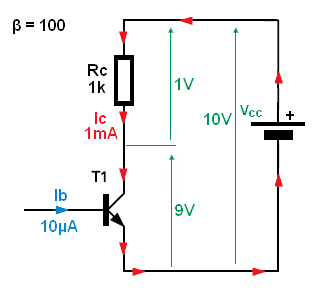# Transistor current gain in saturation mode

Hi, I want to operate my transistor as a switch. I am using this one
Datasheet:
http://www.farnell.com/datasheets/1868820.pdf

In the data sheet, it says the DC current gain hfe = 110 which I am assuming thats for the linear operating region ?, so if my hfe is less than 110 then will it be in saturation ?

I see in one of the Vce(sat) vs Ic graphs that hfe = 10. If my current gain is 20, will it still be in saturation ?

## Answers and Replies

In the data sheet, it says the DC current gain hfe = 110 which I am assuming thats for the linear operating region ?
Yes, in active region (Vce = 5V and Ic = 2mA)

so if my hfe is less than 110 then will it be in saturation
Yes and No, It depends on base current/collector current.For this circuit with ideal transistor (CCCS) any base current large than:
Ib > (Vcc/Rc)/β will saturate our BJT.

But in real life ideal transistor don't exist. For any real world transistor the β is not constant. Beta varies with Ic, Vce, temperature. And what is worse, every single transistor will have different beta value and beta will changes for different operating conditions also.
Also in saturation Ic = Ib * β do not hold any more.
So too overcome this problem with beta and saturation we are forced to use "overdrive factor" or "Forced Beta" trick.
We simply increase the base current well beyond Ib > (Vcc/Rc)/β (beyond minimum beta). We do this to make sure that we have enough base current to put the transistor well into saturation for every condition we have in our circuit.

I see in one of the Vce(sat) vs Ic graphs that hfe = 10. If my current gain is 20, will it still be in saturation ?

Most BJT's vendors define saturation region when Ic/Ib = 10 (called Forced Beta). And the most data-sheet show Vce_sat for Ic/Ib = 10
So to be one hundred percent sure that your BJT will be in saturation you must use this so-called forced beta technique when choosing base resistor value.

Ib/Ic = 10

Rb = (Vin - Vbe)/(0.1*Ic)

Rc = (Vcc - Vce_sat)/Ic

Or

Ib = (Vin - Vbe)/Rb

Vce = Vcc - Ic*Rc = Vcc - hfe*Ib*Rc =Vcc - Hfe*(Vin - Vbe)/Rb*Rc = Vcc - hfe*Rc/Rb * (Vin - Vbe) --> Solving for Rb

$$Rb\leqslant \frac{Vin(min) - Vbe}{Vcc - Vce(sat)}*\frac{hfe(min)}{K}*Rc$$

K = 3...10 - overdrive factor

Last edited:
Svein
Science Advisor
Hi, I want to operate my transistor as a switch. I am using this one
Datasheet:
http://www.farnell.com/datasheets/1868820.pdf
Those transistors are not very good as switches. They will go into saturation willingly enough, but they will take "forever" (meaning at least 100μs) to come out of saturation. A rule of thumb says that the higher β the transistor has, the slower it will be as a switch. If you want a fast switch transistor, take a look at this data sheet: http://www.farnell.com/datasheets/1662493.pdf. The important characteristics for a switching transistor are shown as "Turn on time" and "Turn off time".

Supplementing Jony130`s excellent answer, I like to mention the reasons for the drastic current gain decrease in saturation mode.
As an example, for VBE=0.7 V and VCE=0.2 V we have VBC=+0.5 V, which means: The base-collector pn junction is forward biased with 0.5 V.
As a consequence, the base node is connected to two forward biased pn junctions and the base current will increase correspondingly. At the same time, the collector current is decreased because the portion of the base current going towards the collector (open BC junction) has the opposite direction if compared with the "normal" collector current. Hence, the ratio IC/IB is drastically reduced. The exact value is hard to determine, but for switching applications we are on the safe side assuming IC/IB=10...20.

Thank you all for the information. I simulated the circuit using multisim and my Vce is about 52mV which is good

@Svein, I'm using the transistor provided by my college for free. Although I know transistors are very cheap and I could go and buy the one you listed but for my project fast switching is not really needed so i'll stick to the one I have